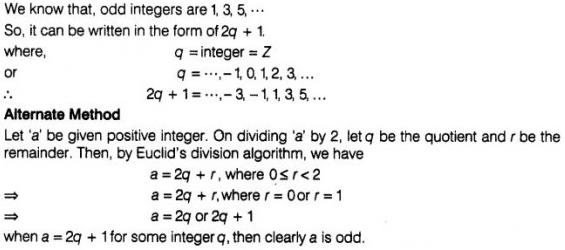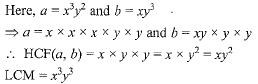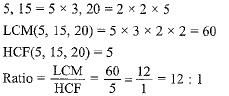Courses

# MCQ : Real Numbers - 1

## 10 Questions MCQ Test Mathematics (Maths) Class 10 | MCQ : Real Numbers - 1

Description
This mock test of MCQ : Real Numbers - 1 for Class 10 helps you for every Class 10 entrance exam. This contains 10 Multiple Choice Questions for Class 10 MCQ : Real Numbers - 1 (mcq) to study with solutions a complete question bank. The solved questions answers in this MCQ : Real Numbers - 1 quiz give you a good mix of easy questions and tough questions. Class 10 students definitely take this MCQ : Real Numbers - 1 exercise for a better result in the exam. You can find other MCQ : Real Numbers - 1 extra questions, long questions & short questions for Class 10 on EduRev as well by searching above.
QUESTION: 1

### √7 is

Solution:

Lets assume that √7 is rational number. ie √7=p/q.
suppose p/q have common factor then
we divide by the common factor to get √7 = a/b were a and b are co-prime number.
that is a and b have no common factor.
√7 =a/b co- prime number
√7= a/b
a=√7b
squaring
a²=7b² .......1
a² is divisible by 7
a=7c
substituting values in 1
(7c)²=7b²
49c²=7b²
7c²=b²
b²=7c²
b² is divisible by 7
that is a and b have atleast one common factor 7. This is contradicting to the fact that a and b have no common factor.This happend because of our wrong assumption.
√7 is irrational

QUESTION: 2

### For positive integers a and 3, there exist unique integers q and r such that a = 3q + r, where r must satisfy:

Solution:

Euclid’s division Lemma:

It tells us about the divisibility of integers. It states that any positive integer ‘a’ can be divided by any other positive integer ‘ b’ in such a way that it leaves a remainder ‘r’.

Euclid's division Lemma states that for any two positive integers ‘a’ and ‘b’ there exist two unique whole numbers ‘q’ and ‘r’ such that , a = bq + r, where 0≤ r < b.

Here, a= Dividend, b= Divisor, q= quotient and r = Remainder.

SOLUTION :

Given : a = 3q+r

In this question ,

b = 3

The values 'r’ can take 0 ≤ r < 3.

QUESTION: 3

### For some integer q, every odd integer is of the form

Solution:QUESTION: 4

Find the greatest number of 5 digits, that will give us remainder of 5, when divided by 8 and 9 respectively.

Solution:

The greatest number will be multiple of LCM (8, 9)
LCM of 8 and 9 = 72
On verification we find that 99941 when divided by 72 leaves remainder 5.

QUESTION: 5

The product of two consecutive integers is divisible by

Solution:

As the case is of 2 consecutive integers, one will be odd and the other one even, so their product will always be even which eventually will be divisible by an even no., i.e. 2, here.

QUESTION: 6

If two positive integers a and b are written as a = x3y2 and b = xy3, where x, y are prime numbers, then LCM(a, b) is

Solution:QUESTION: 7

n2 - 1 is divisible by 8 if n is

Solution:

If n is even then it is not possible for n=2. If n is natural number then it is not possible for n=1,2.If n is integer similarly n²- 1 is not divisible by 8.If n is odd the possible for n is 3,5,7. Thus the n is odd number

QUESTION: 8

If two positive integers p and q can be expressed as p = ab2 and q = a3b; where a, b being prime numbers, then LCM (p, q) is equal to

Solution:

As per question, we have,

p = ab2 = a × b × b

q = a3b = a × a × a × b

So, their Least Common Multiple (LCM) = a3 × b2

QUESTION: 9

If two positive integers a and b are written as a = x3y2 and b = xy3; x, y are prime numbers, then LCM (a, b) is

Solution:

A=x3y2=x(x)(x)(y)(y)
b=xy3=x(y)(y)(y)
LCM of a and b=xy2(x2×y)
=x3y3
so option C is the correct answer

QUESTION: 10

The ratio between the LCM and HCF of 5,15, 20 is:

Solution: